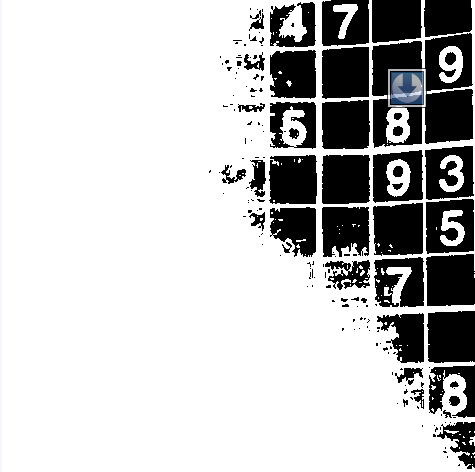## Recognizing digits

Series: SuDoKu Grabber in OpenCV:

1. The Plot
2. Grid detection
3. Extracting the grid
4. Extracting digits
5. Recognizing digits

In the previous article (Detecting a SuDoKu puzzle in an Image, Part 3), we created the DigitRecognizer class.It had functions for training and classifying images as digits. We also put some pre-processing code to ensure the image is at the center. Now, we'll combine it with our existing program. We'll also use the merged lines we created earlier.

## Training the classifier

We'll start where we left the original SuDoKu program. Your last line was probably:

```cv::warpPerspective(original, undistorted, cv::getPerspectiveTransform(src, dst), Size(maxLength, maxLength));
```

Unless you tried hacking a bit yourself!

First thing we'll do is create a thresholded image. Till now, the text is in black. But we want it to be white. So, we'll create a duplicate and use an inverted threshold:

```Mat undistortedThreshed = undistorted.clone();
```

I've used the adaptive threshold (with a block size of 101 to calculate the dynamic threshold value) to keep the effects of lighting from spoiling the thresholding.

Had I used standard thresholding, it would look something like this:With the adaptive thresholding, the thresholded image looks like this:Next, we create an instance of the DigitRecognizer class and train it:

```DigitRecognizer *dr = new DigitRecognizer();
bool b = dr->train("D:/Test/Character Recognition/train-images.idx3-ubyte", "D:/Test/Character Recognition/train-labels.idx1-ubyte");
```

If you want you can put a check on 'b'. If true, the training was successful, otherwise its false.

Then, we create a small image that will hold one SuDoKu cell:

```int dist = ceil((double)maxLength/9);
Mat currentCell = Mat(dist, dist, CV_8UC1);
```

Now, we iterate through each cell:

```for(int j=0;j<9;j++)
{
for(int i=0;i<9;i++)
{
```

Each of these cells correspond to certain pixels. We copy these pixels into currentCell:

```        for(int y=0;y<dist && j*dist+y<undistortedThreshed.cols;y++)
{

uchar* ptr = currentCell.ptr(y);

for(int x=0;x<dist && i*dist+x<undistortedThreshed.rows;x++)
{
ptr[x] = undistortedThreshed.at<uchar>(j*dist+y, i*dist+x);
}
}
```

Once we've copied the pixels, we calculate the number of white pixels on it. If the number is less, it's probably a blank cell. Otherwise, it's probably a numbered cell.

```        Moments m = cv::moments(currentCell, true);
int area = m.m00;
if(area > currentCell.rows*currentCell.cols/5)
{
int number = dr->classify(currentCell);
printf("%d ", number);
}
else
{
printf("  ");
}
```

And finally, we end the loops:

```    }
printf(" ");
}
```

Try running the program. You should see the SuDoKu printed on the console.

## Accuracy

Well. the recognition isn't very accurate. I can think of two main factors:

• The training data is for handwritten text
• The algorithm used isn't great

Other possible things you can use for recognizing the text are neural networks, haar networks or some chain-codes thing (the text on the SuDoKu grid has proper and well defined boundaries).

## Summary

Today, we loaded the DigitRecognizer class into the main program and made it recognize digits. And with that, the series comes to an end! Hope you've learned a lot over the course of this series.

## More in the series

This tutorial is part of a series called SuDoKu Grabber in OpenCV:

1. The Plot
2. Grid detection
3. Extracting the grid
4. Extracting digits
5. Recognizing digits

#### Related posts#### 7 unique neural network architectures